# Nested Recursion

## Nested Recursion in C Language with Examples

In this article, I am going to discuss Nested Recursion in C Language with Examples. Please read our previous article, where we discussed Indirect Recursion in C  with Examples.

##### Nested Recursion in C

In Nested recursion, the recursive function will pass the parameter as a recursive call. For better understanding, please have a look at the below image. In the below image nested is a recursive function which calls itself. But the parameter itself is a recursive call i.e. nested(v-1). This is called nested recursion.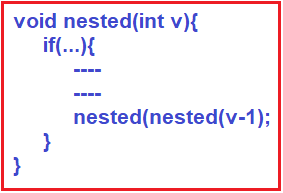##### Example: Nested Recursion in C Language:

In the below example, we have used simple logic to explain how nested recursion works. Here we created a function nested that takes one integer parameter. Inside this function there is an if-else block, it checks if v > 100 then return v- 10 else return nested (nested (v + 11)). Looking complex? Let’s understand this with a step-by-step explanation.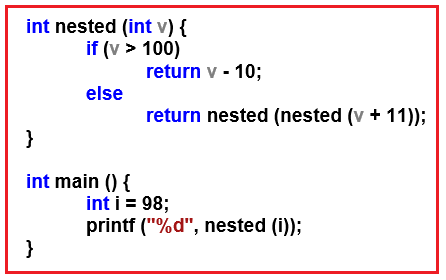##### Tracing in Nested Recursion in C Language:

Let us trace the above example step by step for a better understanding of Nested Recursion.

##### Step1.Tracing Tree: In step1, we call the function nested with a parameter value of 98. Inside this call first, it checks for the condition if 98 > 100? No, then it goes to else part which return nested (nested (98 + 11)) i.e. it is calling the fun function with increased value of v i.e. 109 (98 + 11)

Nested Call: On the right-hand side of the above diagram, we have explained how nested (nested (109)) will be calculated. Now it will call the fun function with the 109. Again, it will check if 109 > 100? Yes, then it returns 99(109 – 10).

##### Step2: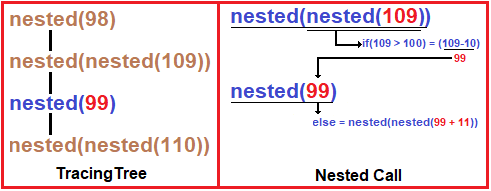Tracing Tree: Now nested (99) has to be calculated.

Nested Call: Again, it checks for conditions if 99 > 100? No, then it goes to the else part which returns nested (nested (99 + 11)).

##### Step3: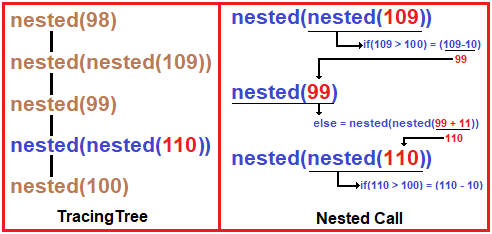Tracing Tree: Now nested (nested (110)) executes.

Nested Call: In nested (nested (110)), first, the compiler will calculate nested (110). It first checks if 110 > 100? Yes, then returns 100(110 – 10), then control goes to nested (100).

##### Step4: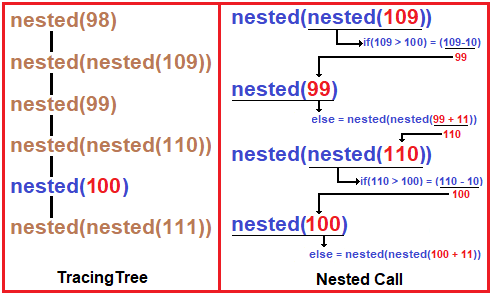Tracing Tree: Now nested (100) executes.

Nested Call: In nested (100), it first checks if 100 > 100? No, then it goes to the else part which returns nested (nested (100 + 11).

##### Step5: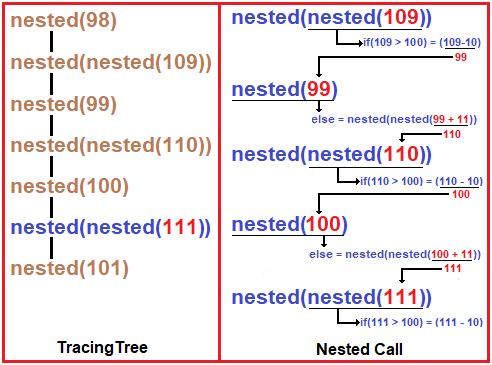Tracing Tree: Now nested (nested (111)) executes.

Nested Call: In nested (nested (111)), first, the compiler will calculate nested (111). It first checks if 111 > 100? Yes, then returns 101(111 – 10), then control goes to nested (101).

##### Step6: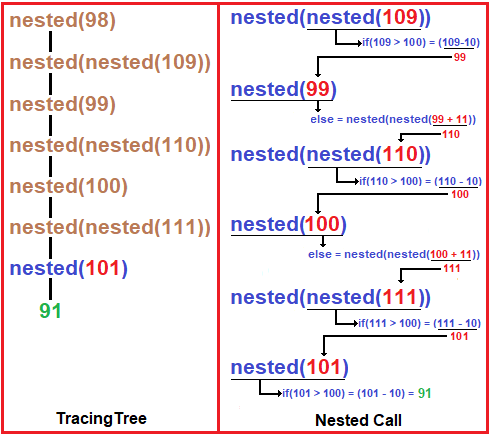Tracing Tree: Now nested (101) executes.

Nested Call: In nested (101), it first checks if 101 > 100? Yes, then it will return 91(101 – 10).

##### Step7: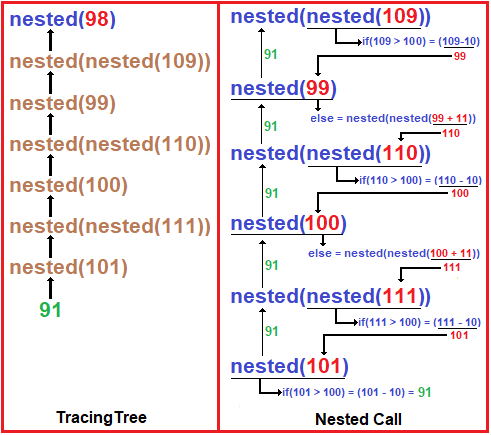Tracing Tree: From here 91 will return to previous recursive calls as shown above in the diagram.

Nested Call: Now all calls of the nested (98) function have finished.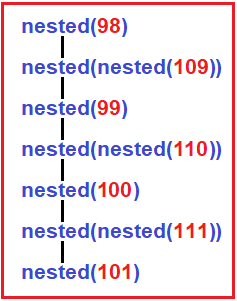The above diagram shows the complete version of the Tracing Tree.

##### Complete Example Code of Nested Recursion in C Language:
```#include <stdio.h>
int nested (int v) {
if (v > 100)
return v - 10;
else
return nested (nested (v + 11));
}

int main () {
int i = 98;
printf ("%d", nested (i));
}
```

Output: 91

In the next article, I am going to discuss the Sum of Natural numbers in the C Language with Examples. Here, in this article, I try to explain Nested Recursion in C Language with Examples and I hope you enjoy this Indirect Recursion in C Language with Example article.